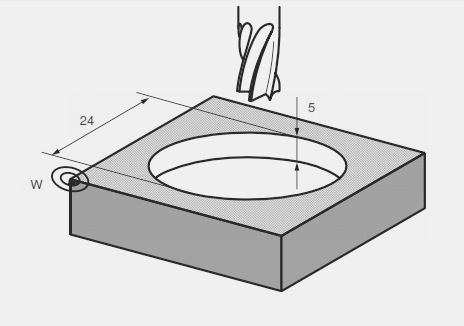# Program Example L930 Milling Circular Pocket Sinumerik 840C

For cnc machinists who work on Siemens Sinumerik 840 840C controls, here is a program example to mill a circular pocket on their cnc milling machines.

Although Sinumerik L930 Milling Circular Pocket cycle has many R parameters, most of them are self explanatory you might find a brief articles about L930 cycle here L930 Milling Circular Pocket – Sinumerik 840 840C Programming.

## L930 Milling Circular Pocket CNC Program ExampleL930 Milling Circular Pocket

```N10 G90 G0 X50 Y30 Z20 D05 T04 S600 M03
N20 R1=2.5 R2=2 R3=-5 R6=3
R15=300 R16=100 R22=50 R23=30
R24=12 L930 P1
N30 Z50
N40 M30```